Technical Article

# Learn About the Basics of LVDT Demodulator Circuits

May 16, 2021 by Dr. Steve Arar

## Learn where demodulator circuits are needed and how you can convert the AC output of an LVDT (linear variable differential transformer) into DC signals using a diode rectifier.

In a previous article, we looked at the structure and operation of an LVDT sensor.

In this article, we’ll see how a diode rectifier can be used as a demodulator circuit to convert the AC outputs of an LVDT into a useful DC signal that indicates the core position.

### The Need for a Demodulator

When the core of an LVDT is perfectly centered, equal voltages with opposite polarities appear across the two secondary windings Vs1 = -Vs2 and Vout = 0.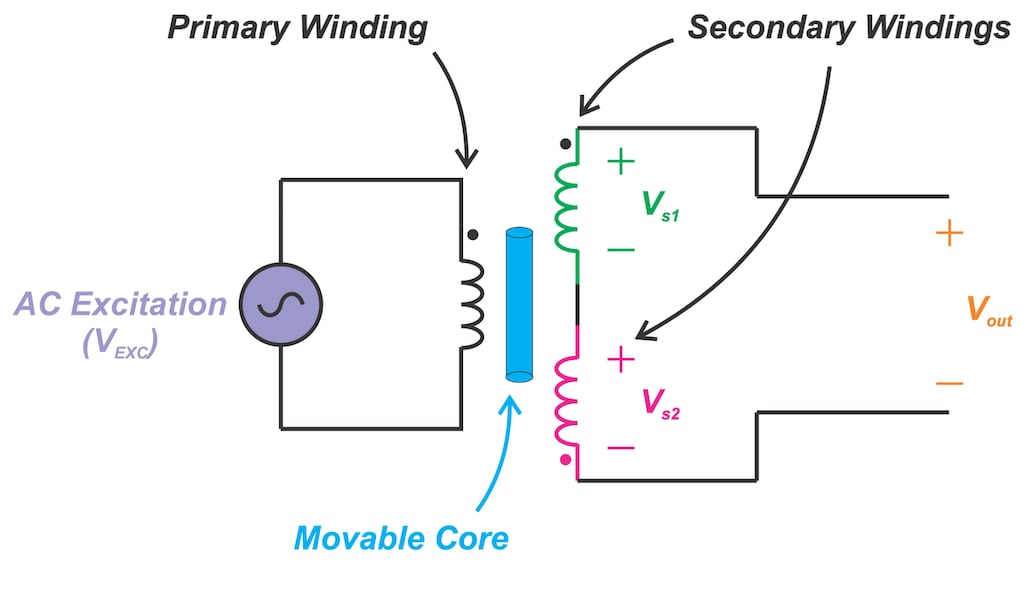##### Figure 1

As the core is moved off the center in a given direction, the voltage across one of the secondary coils increases and the other one decreases linearly with the core displacement, and consequently, the amplitude of Vout increases. If we convert Vout into a DC signal, we can determine the amount of the core displacement.

However, without knowing the phase of Vout with respect to the excitation voltage (VEXC), we cannot determine in which direction the core is displaced. Hence, we need some circuitry to successfully interpret the LVDT output to determine both the amount of the displacement and the direction in which the core is displaced.

In the context of the LVDT signal conditioning, a demodulator is the circuit that converts the AC output of the LVDT to a DC signal whose amplitude and polarity reveals the core position. Rectification-based demodulators and synchronous demodulators are the two main options that can be used with an LVDT device.

### Diode Half-Wave Rectifier

A diode half-wave rectifier, shown in Figure 2, can be used as an LVDT demodulator.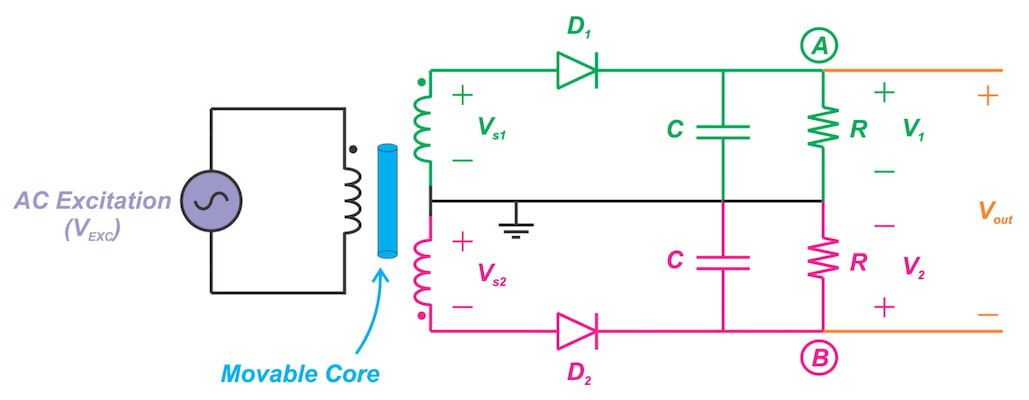##### Figure 2

The voltage from the first secondary (Vs1) is rectified by means of the half-wave rectifier created by D1 and the upper R and C. Similarly, the rectified version of Vs2 appears at node B. The output is the difference between these two DC voltages, i.e., Vout = V- V2

### Examining the Waveforms of a Diode Demodulator

To gain a deeper insight, let’s do some simulations and examine the operation of a diode demodulator. Assume that we have VEXC = 4sin(2π x 2500 x t). Moreover, suppose that, at the null position, the amplitude of both Vs1 and Vs2 is equal to 4 V; however, due to a given core displacement, the voltages across the two secondaries changes to:

Vs1 = 4 x (1+0.3) x sin(2π x 2500 x t)

##### Equation 1

Vs2 = -4 x (1-0.3) x sin(2π x 2500 x t)

##### Equation 2

Here, we are assuming that the amplitude of the first secondary has increased by 1.2 V compared to the null position amplitude; and the amplitude of the second secondary has reduced by the same value (1.2 V). We can use the following LTspice schematic to simulate this example: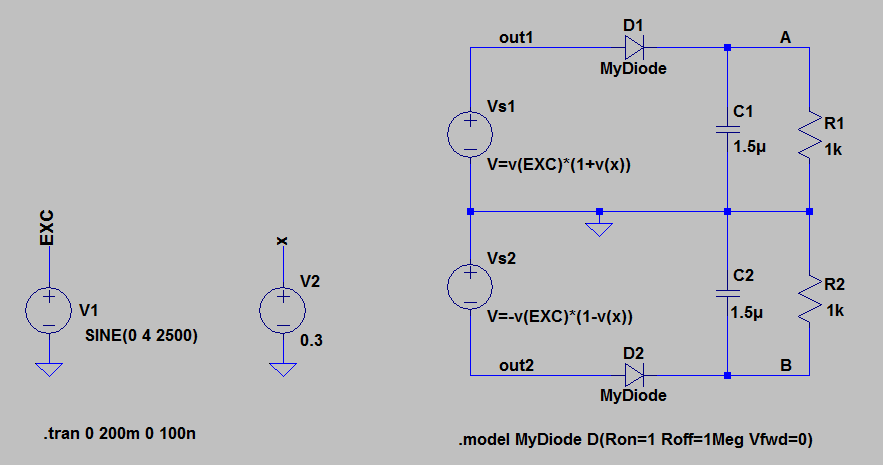##### Figure 3

In this schematic, the voltage sources Vs1 and Vs2 are LTspice “arbitrary behavioral voltage sources” that are used to create the voltages given by Equations 1 and 2. For example, Vs1 is equal to the voltage at node EXC, v(EXC), multiplied by the factor 1 plus the voltage at node x, i.e. 1+v(x). The voltage of node EXC is the excitation voltage and the voltage at node x is 0.3. This gives Vs1 = v(EXC) x (1+0.3) = (1+0.3) x 4 x sin(2π x 2500 x t) that is the same as Equation 1.

The diodes D1 and D2 are ideal diodes defined by the LTspice .model statement. With R=1 kΩ and C=1.5 μF, we get the following waveforms for the upper half-wave rectifier: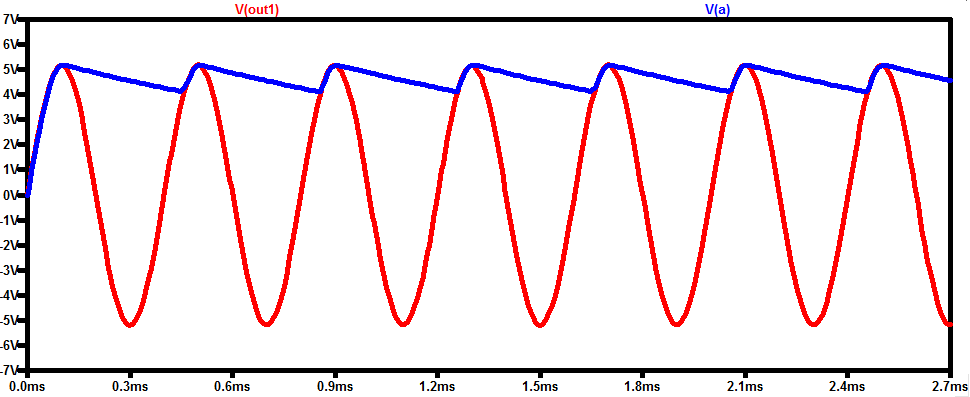##### Figure 4

Ignoring the voltage ripples, node A has a DC value of about 4.66 V. For the lower rectifier, we get the following waveforms.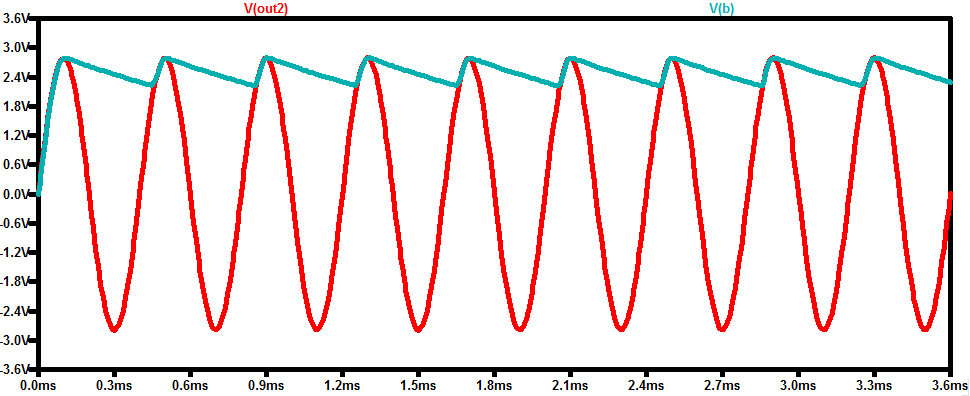##### Figure 5

The DC value of node B is smaller as expected (about 2.51 V). The output is the difference between these two DC voltages and has a DC value of about 2.15 V. The magnitude of the output is proportional to the amount of the core displacement. Considering the polarity of the output, we know that |Vs1| > |Vs2|. This reveals the direction in which the core is displaced.

### Simulating a System With a Mechanical Bandwidth of 250 Hz

Now, let’s examine the above system under the assumption that the motion of the object attached to the core has a sinusoidal waveform at 250 Hz:

Core Motion = Maximum Displacement x sin(2π x 250 x t)

Since the amplitudes of the LVDT outputs vary linearly with the core position, we conclude that Vs1 and Vs2 can be represented by the following equations:

Vs1 = 4 x (1+x) x sin(2π x 2500 x t)

##### Equation 3

Vs2 = -4 x (1-x) x sin(2π x 2500 x t)

##### Equation 4

where x is a sinusoidal at 250 Hz. Assume that, for a given LVDT, the amplitude of x is 0.3. Hence, we have

x = 0.3 x sin(2π x 250 x t)

We can use the following LTspice schematic to simulate this example: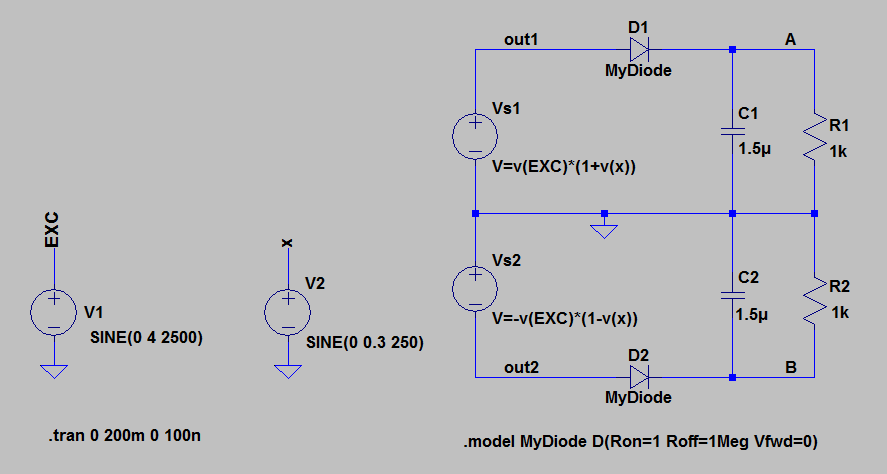##### Figure 6

This is the same as the previous example except that the change in the amplitude of Vs1 and Vs2 follows a sinusoidal waveform (v(x)=0.3×sin(2π×250×t)). The voltages at nodes out1 and A are shown below.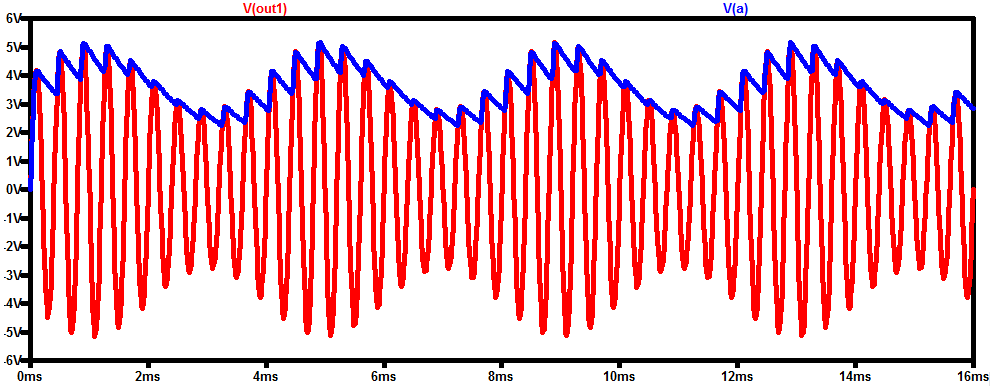##### Figure 7

As you can see, the voltage across the secondary is a sinusoidal waveform whose amplitude is modulated by the core position (In our simulation, the amplitude is actually modulated by x, which is assumed to be a function of the core position). This explains why the circuitry used to extract the core position information is called a demodulator.

For the lower rectifier, we get similar waveforms as shown in Figure 8.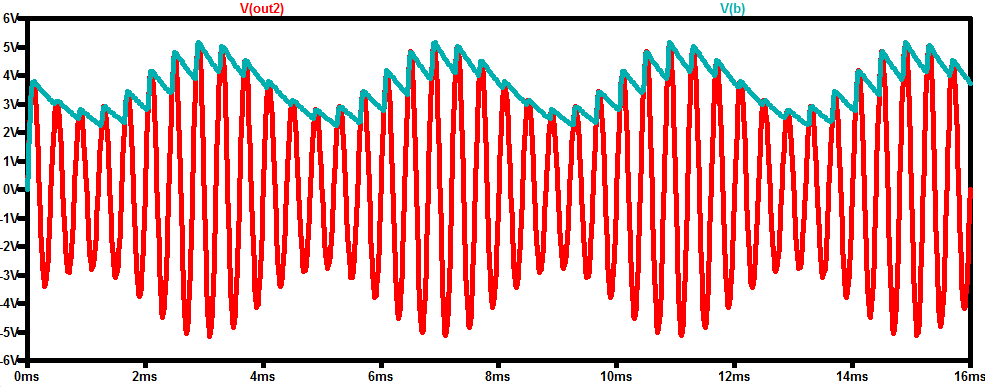##### Figure 8

The red curve in the following figure shows the final output (Vout = V(a)-V(b)).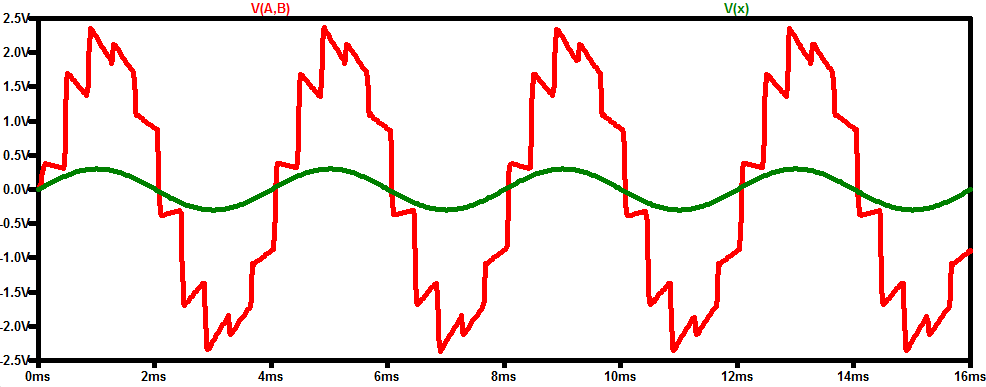##### Figure 9

Although the output signal has some abrupt changes, it looks like the amplified version of x which is a function of the core displacement.

Hence, it seems that the modulator output gives us the core position as expected. To verify this, we can use the FFT feature of the LTspice to find the frequency content of the output voltage. This is shown in Figure 10.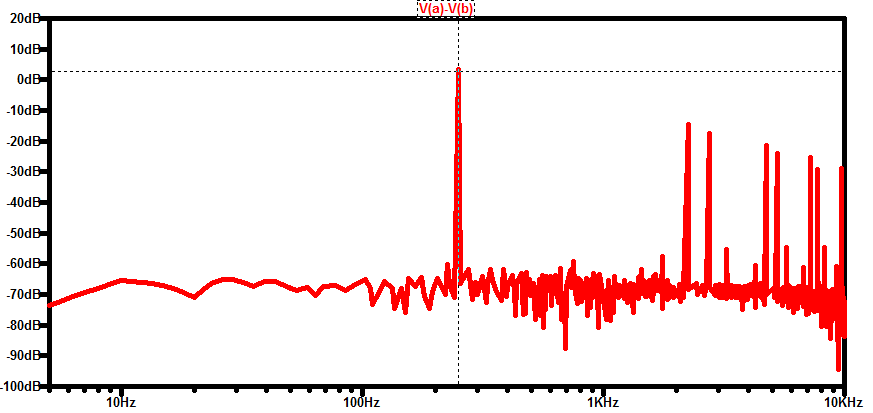##### Figure 10

The output FFT shows that the main frequency component is at 250 Hz which is the frequency of the object motion. There are also some high-frequency components that can be filtered by a low-pass filter in the subsequent stages of the signal conditioning circuitry.

### Limitations of the Diode Half-Wave Rectifier

The above simulations incorporate an ideal diode model. Real-world diodes exhibit a non-zero forward voltage drop. This can lead to non-linearity error for the cases in which the amplitude of the LVDT outputs are relatively small. To avoid the non-linear region of the diode I-V characteristic, the amplitude of the LVDT secondaries should be larger than the forward voltage drop of the diodes even when the core is at its maximum distance from the null position.

Remember that with the core at its full-scale displacement, the voltage across one of the secondaries is at its minimum. With some miniature and specialty LVDTs, the output amplitude might be relatively small and the diode forward voltage can cause issues.

Moreover, the forward voltage drop of a diode is a function of temperature (with a temperature coefficient of about -2.2 mV/°C for silicon). The forward voltage drop can even change with mechanical stress caused by the soldering process. Another mechanism that can lead to mechanical stress is the difference in thermal coefficients of expansion between the diode bodies and the circuit board. Hence, it can be challenging to have sufficiently matched rectifiers for the two LVDT outputs.

In addition to the forward voltage drop of the diodes, the impedance of the two paths should also be matched to avoid unwanted mismatch between the response of the two secondaries.

### Precision Rectifier

To circumvent the limitations of the diode rectifier, we can use a precision rectifier, shown in Figure 11, to obtain the DC value of each of the LVDT secondaries.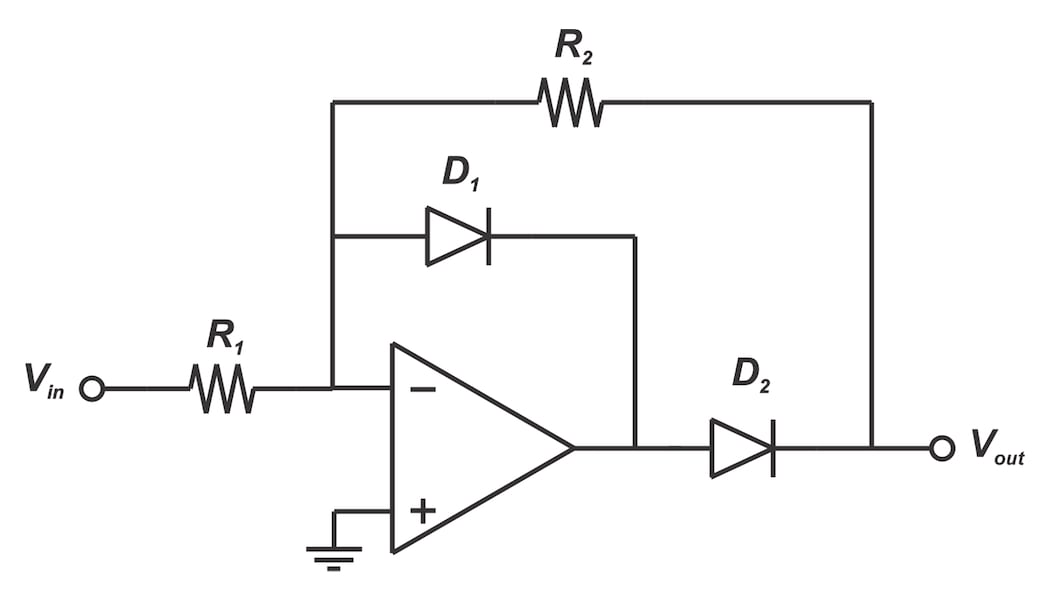##### Figure 11

Although a precision rectifier can remedy the challenges of a simple diode rectifier, it has its own limitations such as little noise rejection. In the next article, we’ll look at this circuit in greater detail and discuss synchronous demodulators for the LVDT applications.